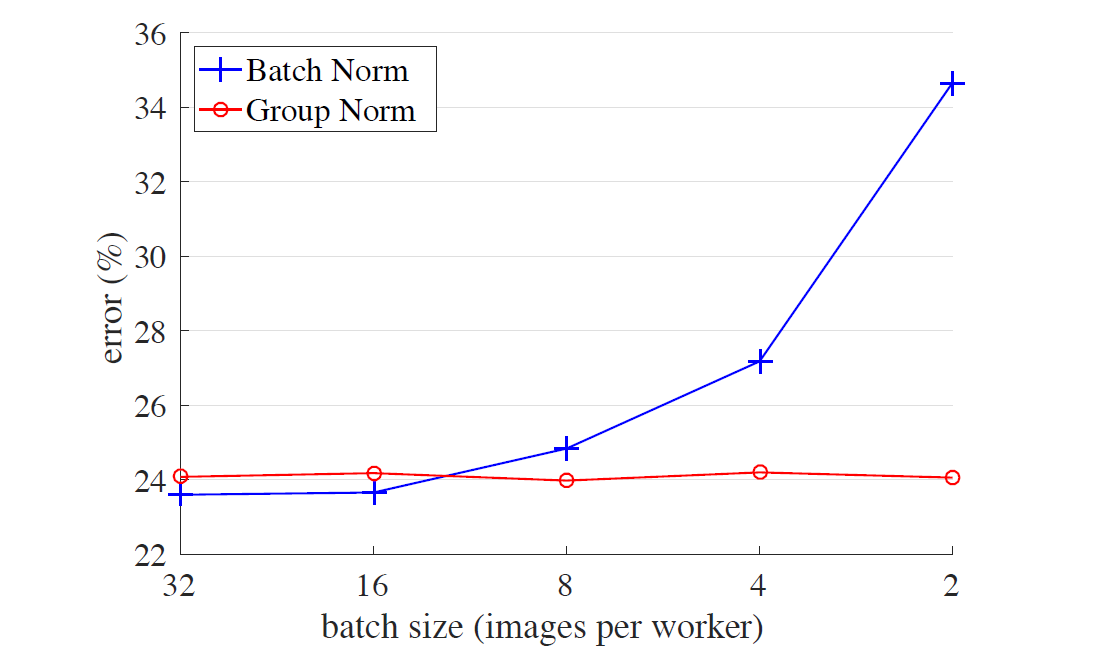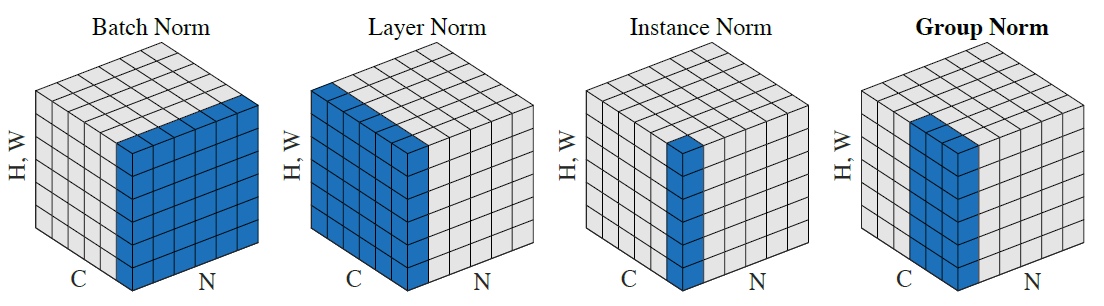Jarvis' Blog (总有美丽的风景让人流连) 总有美丽的风景让人流连

# Group Normalization 阅读笔记

2018-04-10
Jarvis
Post

## 前言• 批标准化 (Batch Norm, BN)
• 层标准化 (Layer Norm, LN)
• 实例标准化 (Instance Norm, IN)
• 权重标准化 (Weight Norm)## Group Normalization

$\mu_i = \frac1m\sum_{k\in \mathcal{S}_i}x_k, \qquad \sigma_i = \sqrt{\frac1m\sum_{k\in \mathcal{S}_i}(x_k - \mu_i)^2 + \epsilon},$

Batch Norm $$\mathcal{S}_i: \{k\lvert k_C = i_C\}$$ $$i_C$$(和 $$k_C$$) 表示沿着 $$C$$ 轴的指标. 左式的意思是同一个通道的像素在一起标准化. 即对于每个通道, BN 沿着 $$(N, H, W)$$ 轴计算 $$\mu$$ 和 $$\sigma$$ , 得到的形状为 $$C$$ .
Layer Norm $$\mathcal{S}_i: \{k\lvert k_N = i_N\}$$ 对于每个样本, LN 沿着 $$(C, H, W)$$ 轴计算 $$\mu$$ 和 $$\sigma$$ , 得到的形状为 $$N$$.
Instance Norm $$\mathcal{S}_i: \{k\lvert k_N = i_N, k_C = i_C\}$$ 对每个样本每个通道, IN 沿着 $$(H, W)$$ 轴计算 $$\mu$$ 和 $$\sigma$$ , 得到的形状为 $$N\times C$$.
Group Norm $$\mathcal{S}_i: \{k\lvert k_N = i_N, \lfloor\frac{k_C}{C/G}\rfloor = \lfloor\frac{i_C}{C/G}\rfloor\}$$ $$G$$ 表示分组的数量, 是一个预定义的超参数(一般选择 $$G=32$$). $$C/G$$ 是每个组的通道数, 第二个等式条件表示对处于同一组的像素计算均值和标准差. 最终得到的形状为 $$N\times (C/G)$$ .

$y_i= \gamma\hat{x_i} + \beta$

### 实现

1
2
3
4
5
6
7
8
9
10
11
12
13
14
15
16
17
18
19
def GroupNorm(x, gamma, beta, G, eps=1e-5):
""" Implementation of group normalization

Params
------
x: input features with shape [N, C, H, W]
gamma, beta: scale and shift, with shape [1, C, 1, 1]
G: number of groups for GN
"""
assert C % G == 0, "C should be divisible by G"

N, C, H, W = x.shape.as_list()
x = tf.reshape(x, [N, G, C//G, H, W])

mean, var = tf.nn.moments(x, [2, 3, 4], keep_dims=True)
x = (x - mean) / tf.sqrt(var + eps)
x = tf.reshape(x, [N, C, H, W])

return x * gamma + beta


Group Normalization

Content